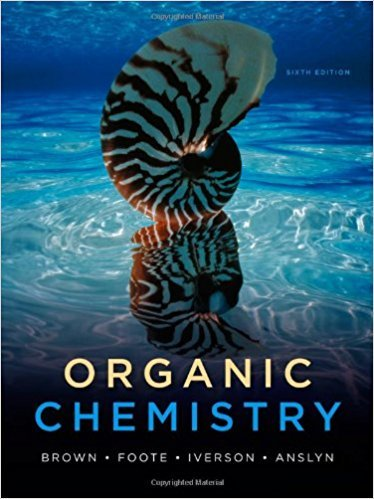×
×

# Solutions for Chapter 22: Organic Chemistry 6th Edition## Full solutions for Organic Chemistry | 6th Edition

ISBN: 9780840054982Solutions for Chapter 22

Solutions for Chapter 22
4 5 0 317 Reviews
23
1
##### ISBN: 9780840054982

Chapter 22 includes 62 full step-by-step solutions. This textbook survival guide was created for the textbook: Organic Chemistry, edition: 6. This expansive textbook survival guide covers the following chapters and their solutions. Since 62 problems in chapter 22 have been answered, more than 33881 students have viewed full step-by-step solutions from this chapter. Organic Chemistry was written by and is associated to the ISBN: 9780840054982.

Key Chemistry Terms and definitions covered in this textbook
• aqueous solution.

A solution in which the solvent is water. (4.1)

• continuous-wave (CW) spectrometer

An NMR spectrometer that holds the magnetic field constant and slowly sweeps through a range of rf frequencies, monitoring which frequencies are absorbed.

• copolymer.

A polymer containing two or more different monomers. (25.2)

• critical temperature

The highest temperature at which it is possible to convert the gaseous form of a substance to a liquid. The critical temperature increases with an increase in the magnitude of intermolecular forces. (Section 11.4)

• diamagnetic anisotropy

An effect that causes different regions of space to be characterized by different magnetic field strengths.

• divalent

An element that forms two bonds, such as oxygen.

• enantiomeric excess

For a mixture containing two enantiomers, the difference between the percent concentration of the major enantiomer and the percent concentration of its mirror image.

• frontier orbitals

The highest occupied molecular orbital (HOMO) and lowest unoccupied molecular orbital (LUMO) that participate in a reaction.

• gas constant (R)

The constant of proportionality in the ideal-gas equation. (Section 10.4)

• Gibbs free energy

A thermodynamic state function that combines enthalpy and entropy, in the form G = H - TS. For a change occurring at constant temperature and pressure, the change in free energy is ?G = ?H - T?S. (Section 19.5)

• glucose

A polyhydroxy aldehyde whose formula is CH2OH1CHOH24CHO; it is the most important of the monosaccharides. (Section 24.8)

• High-density lipoprotein (HDL)

Plasma particles, density 1.06–1.21 g/mL, consisting of approximately 33% proteins, 30% cholesterol, 29% phospholipids, and 8% triglycerides.

• Imine

A compound containing a carbon-nitrogen double bond, R2C"NR’; also called a Schiff base

• Keq

A term that describes the position of equilibrium for a reaction: Keq = 3H3O+ 4 3A- 4 3HA4 3H2O4

• Mercaptan

A common name for a thiol; that is, any compound that contains an -SH (sulfhydryl) group

• Meta (m)

Refers to groups occupying 1,3-positions on a benzene ring.

• molarity

The concentration of a solution expressed as moles of solute per liter of solution; abbreviated M. (Section 4.5)

• molecular solids

Solids that are composed of molecules. (Sections 12.1 and 12.6)

A chemical entity with an unpaired electron.

• rate-determining step

The sloweststep in a multistep reaction which determines the rate of the reaction.

×